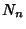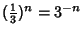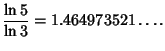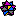## Box FractalA Fractal which can be constructed using String Rewriting by creating a matrix with 3 times as many entries as the current matrix using the rulesLetbe the number of black boxes,the length of a side of a white box, andthe fractional Area of black boxes after theth iteration.(1)(2)(3)

The Capacity Dimension is therefore(4)Weisstein, E. W. Fractals.'' Mathematica notebook Fractal.m.